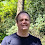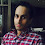### Overview

Many developers have determined that BigDecimal is the only way to deal with money.  Often they site that by replacing double with BigDecimal, they fixed a bug or ten.  What I find unconvincing about this is that perhaps they could have fixed the bug in the handling of double and that the extra overhead of using BigDecimal.

My comparison, when asked to improve the performance of a financial application, I know at some time we will be removing BigDecimal if it is there. (It is usually not the biggest source of delays, but as we fix the system it moves up to the worst offender)

### BigDecimal is not an improvement

BigDecimal has many problems, so take your pick, but an ugly syntax is perhaps the worst sin.
• BigDecimal syntax is an unnatural.
• BigDecimal uses more memory
• BigDecimal creates garbage
• BigDecimal is much slower for most operations (there are exceptions)
The following JMH benchmark demonstrates two problems with BigDecimal, clarity and performance.

The core code takes an average of two values.

The double implementation looks like this.  Note: the need to use rounding.

mp[i] = round6((ap[i] + bp[i]) / 2);

The same operation using BigDecimal is not only long, but there is lots of boiler plate code to navigate

.divide(BigDecimal.valueOf(2), 6, BigDecimal.ROUND_HALF_UP);

Does this give you different results?  double has 15 digits of accuracy and the numbers are far less than 15 digits.  If these prices had 17 digits, this would work, but nor work the poor human who have to also comprehend the price (i.e. they will never get incredibly long)

### Performance

If you have to incurr coding overhead, usually this is done for performance reasons, but this doesn't make sense here.

Benchmark                          Mode Samples Score  Score error    Units
o.s.MyBenchmark.bigDecimalMidPrice thrpt  20    23638.568  590.094    ops/s
o.s.MyBenchmark.doubleMidPrice     thrpt  20   123208.083 2109.738    ops/s

### Conclusion

If you don't know how to use round in double, or your project mandates BigDecimal, then use BigDecimal.  But if you have choice, don't just assume that BigDecimal is the right way to go.

### The code

import org.openjdk.jmh.annotations.Benchmark;
import org.openjdk.jmh.annotations.Scope;
import org.openjdk.jmh.annotations.State;
import org.openjdk.jmh.runner.Runner;
import org.openjdk.jmh.runner.RunnerException;
import org.openjdk.jmh.runner.options.Options;
import org.openjdk.jmh.runner.options.OptionsBuilder;

import java.math.BigDecimal;
import java.util.Random;

public class MyBenchmark {
static final int SIZE = 1024;
final double[] ap = new double[SIZE];
final double[] bp = new double[SIZE];
final double[] mp = new double[SIZE];

final BigDecimal[] ap2 = new BigDecimal[SIZE];
final BigDecimal[] bp2 = new BigDecimal[SIZE];
final BigDecimal[] mp2 = new BigDecimal[SIZE];

public MyBenchmark() {
Random rand = new Random(1);
for (int i = 0; i < SIZE; i++) {
int x = rand.nextInt(200000), y = rand.nextInt(10000);
ap2[i] = BigDecimal.valueOf(ap[i] = x / 1e5);
bp2[i] = BigDecimal.valueOf(bp[i] = (x + y) / 1e5);
}
doubleMidPrice();
bigDecimalMidPrice();
for (int i = 0; i < SIZE; i++) {
if (mp[i] != mp2[i].doubleValue())
throw new AssertionError(mp[i] + " " + mp2[i]);
}
}

@Benchmark
public void doubleMidPrice() {
for (int i = 0; i < SIZE; i++)
mp[i] = round6((ap[i] + bp[i]) / 2);
}

static double round6(double x) {
final double factor = 1e6;
return (long) (x * factor + 0.5) / factor;
}

@Benchmark
public void bigDecimalMidPrice() {
for (int i = 0; i < SIZE; i++)
.divide(BigDecimal.valueOf(2), 6, BigDecimal.ROUND_HALF_UP);
}

public static void main(String[] args) throws RunnerException {
Options opt = new OptionsBuilder()
.include(".*" + MyBenchmark.class.getSimpleName() + ".*")
.forks(1)
.build();

new Runner(opt).run();
}
}

1.Exactly my point, but not everyone agrees, see the lengthy discussion below http://stackoverflow.com/a/5886596/581205

1.I know that BigDecimal works best for some teams, esp if they don't know how to use double with rounding correctly, but it's developer who act like BigDecimal is the only answer when trading and financial systems predate the use of BigDecimal. Many systems use C++ without decimal.

2.It looks like you are going against Joshua Bloch's recommendation in Effective Java Item 48: 'Avoid float and double if exact answers are required'. It states that 'the float and double types are particularly ill-suited for monetary calculations' and his examples show that rounding doesn't always work. I'd be interested to see you take his examples and make them work using doubles.

1.I've found there a single example (candy buying) and it only shows that comparing doubles is tricky, too. I'm perfectly sure, it can be fixed in one minute. Something line `funds >= price - 0.005` should do. Also `round2(funds) >= round2(price)` should work, albeit slower. Can you give me more examples?

Actually, I'd rather say that monetary calculations are particularly ill-suited for contemporary hardware, but only if *artificially* exact results are required. If you can't tolerate an error of one cent when calculating your country's GDP, then you might be out of luck with double.

3.For monetary calculations long values in cents will work even faster and more predictable, unless your application has to deal with values like US debt.

I share Fadh's concerns regarding "correct rounding": doubles can accomodate far larger values than longs in the same 8 bits due to the partial loss of the precision. And I doubt that any rounding magic will hide this fact in the general case.

1.The US national debt is an estimate, but double and long can support such a number and still have digits of precision left.

4.At my firm I advise teams to use BigDecimal with two exceptions: performance and memory. The example I usually give is matrix calculations for large models: these are both time and space constrained.

It is costly and difficult to have sufficient code inspection to check for proper per-currency rounding using floating point. For example, counterparty reconciliation requires extensive downstream heuristics to match figures with other firms exactly because of inconsistent floating point rounding (sadly, I wrote some of those bugs).

In most of our applications accuracy is more important, there isn't enough data or complex models to get the benefits of floating point. Those applications which need it, generally have teams which recognize when to drop BigDecimal; most teams who need BigDecimal don't realize it and spend time tracking down rounding errors.

So on the balance I agree with your post if you add a few more caveats.

5.Brian,
[quote]At my firm I advise teams to use BigDecimal with two exceptions: performance and memory. The example I usually give is matrix calculations for large models: these are both time and space constrained.[/quote]
Agree, I would even implement matrix calculations with OpenCL on GPU using JavaCL -- this expects double/float as the only option for data types, but it is orders of magnitude more efficient.

Peter,
If you take a look at the upcoming Java Money API (JSR354) then you may notice that there are two implementations of Money in RI -- one "regular" using BigDecimal and one "fast" using long -- see here https://github.com/JavaMoney/jsr354-ri/tree/master/src/main/java/org/javamoney/moneta.

Oracle still avoids itself and do not advise to others to play with rounding of doubles for monetary calculations, even if double has seemingly enough digits of precision...

1.The biggest disadvantage of using long alone is having to keep track of the precision, however if you have a simple library to do this for you, you might get something more natural and faster than BigDecimal. Note: in Java 7+, BigDecimal uses a long when a BigInteger is not required.

6.That round6(double) implementation is non-trivial. It must have taken years of experience to come up with such elegant solution...

7.Heard of http://www.apfloat.org/apfloat_java/? It's LGPL though.